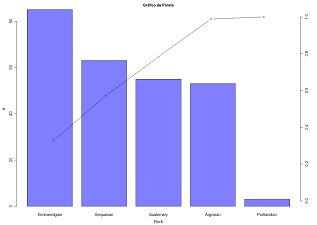## 3. Univariate data analysis

 3.1 Frequency table and histogram (download) Compute a frequency table of a quantitative variable and plot a simple histogram and the cumulative histogram. Classes are of equal interval. Variables to customize: `varlistindex <- 3 # index of the GTD frame variablenclasses <- 10 # number of histogram classes ndecimals <- 3 # number of decimal placeslinedensity <- FALSE # add line of density tracesdensity <- FALSE # add traces of density below histogramabsfrequency <- FALSE # absolute or relative frequenciescolour <- rgb(0,0,1,0.5) # histogram color (0 to 1) levels of red, green, blue and transparency`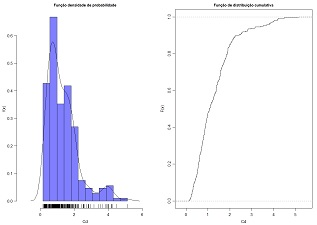3.2 Equal frequency table and histogram (download) Plot a simple histogram of equal frequencies classes and the cumulative histogram. Variables to customize: `varlistindex <- 11 # index of the GTD frame variablenclasses <- 12 # number of histogram classesndecimals <- 3 # number of decimal placescolour <- rgb (1,1,0,0.5) # histogram color (0 to 1) levels of red, green, blue and transparency`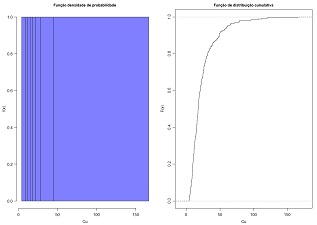3.3 Matrix of histograms (download) Plot a matrix of histograms of a set of quantitative variables. Variables to customize: `nclasses <- 10 # number of histogram classesvarlistindex <- c(5:11) # indexes of the GTD frame variables linedensity <- FALSE # add line of density absfrequency <- TRUE # absolute or relative frequenciestitleGRAPH <- "Matriz de histogramas" # title of the graphiccolour <- rgb(0,0,1,0.5) # histogram color (0 to 1) levels of red, green, blue and transparencypar(mfrow=c(2,4),mar=c(3,3,1,1), oma=c(1,1,2,1), mgp=c(2.0, 0.8, 0))  # graphical window configuration, the first two numbers are the number of rows and columns of the matrix `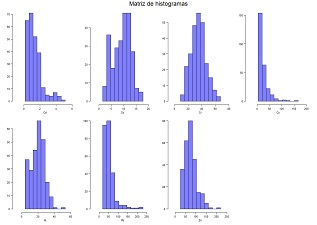3.4 Box plot (download) Draw one or several box-plots in the same graphic. Variables to customize: `varlistindex <- c(5:11) # indexes of the GTD frame variablesplotlog <- FALSE # log scale (FALSE or TRUE)titleGRAPH <- "Box-plots das variáveis em estudo" #title of the graphic`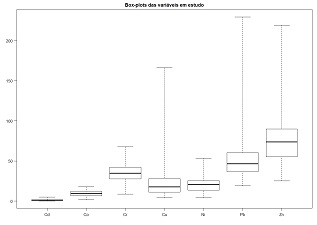3.5 Table of univariate statistics (download) Compute and display several univariate and basic statistics for one or several quantitative variables. The complete list is: minimum, maximum, mode, mean, median, Q1, Q3, variance, standard deviation, variation coeficients, IQR, range, skewness coeficient, Galton coeficient and kurtosis. Variables to customize: `varlistindex <- c(5:11) # indexes of the GTD frame variablesndecimals <- 3 # number of decimal places`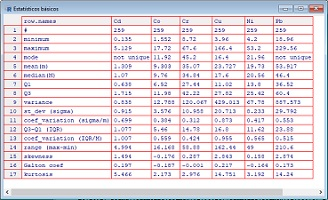3.6 Table of outliers (download) Compute and display the intervals and the number of individuals of the 1st and 2nd order outliers (left and right) for one or several quantitative variables. Variables to customize: `varlistindex <- c(5:11) # indexes of the GTD frame variablesndecimals <- 3 # number of decimal places`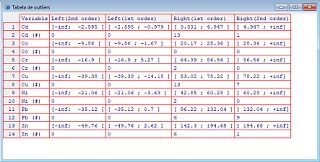3.7 Histogram and box plot (download) Display a simple histogram, a cumulative histogram and the box-plot of one quantitative variable. The box-plot is aligned with the cumulative histogram. Variables to customize: `nclasses <- 10 # number of histogram classesvarlistindex <- 5 # index of the GTD frame variableabsfrequency <- TRUE # absolute or relative frequenciescolour <- rgb(0,0,1,0.5) # histogram color (0 to 1) levels of red, green, blue and transparency`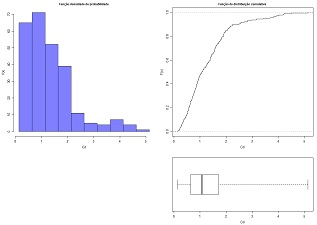3.8 Two overlapped histograms (download) Plot two histograms of two quantitative variables in the same graphic for sake of comparision. Variables to customize: `nclasses <- 20 # number of histogram classesvarlistindex <- c(10,11) # index of the two GTD frame variablevarunits <- c("(ppm)","(ppm)") #units of the variableslinedensity <- FALSE  # add line of density titleGRAPH <- "Histograms" # global titlemaxY <- 0.025 # maximum frequency of the graphiccolour <- c(rgb(0,0,1,0.5),rgb(1,0,0,0.5)) # histogram colors (0 to 1) levels of red, green, blue and transparency (two colours)`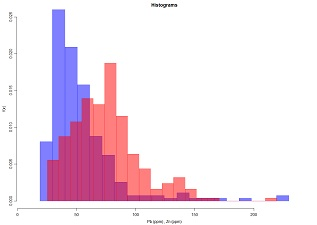3.9 Stem and leaf plot (download) Draw a stem and leaf plot. Variables to customize: `varlistindex <- 11 # index of the GTD frame variable`3.10 Bar diagram and/or pie chart for qualitative variables (download) Plot a bar chart or pie chart for one qualitative variable. Variables to customize: `histtype <- 2 # option for Pie (1) or Bars (2)varlistindex <- 4 # index of the GTD frame qualitative variablecolour <- rgb(0,0,1,0.5) # histogram colors (0 to 1) levels of red, green, blue and transparency (for bars only)`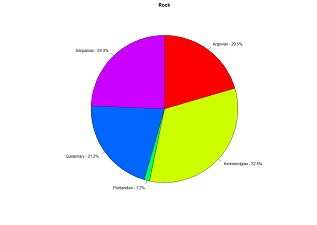3.11 Arithmetic, harmonic and geometric means (download) Compute and compare arithmetic mean, harmonic mean and geometric mean. Variables to customize: `varlistindex <- 11 # index of the GTD frame variablendecimals <- 3 # number of decimal places`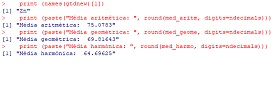3.12 Pareto chart (download) Draw a Pareto chart of a qualitative variable. Variables to customize: `varlistindex <- 4 # index of the GTD frame variabletitleGRAPH <- "Gráfico de Pareto" # global title of the Pareto chartcolour <- rgb(0,0,1,0.5) # histogram colors (0 to 1) levels of red, green, blue and transparency`